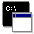## Firefly and PC GAMESS-related discussion club

Learn how to ask questions correctlyRe: Scan along 2 coordinates: how to make both coordinates change at each step of scan?

Alex Granovsky
gran@classic.chem.msu.su

Hello,

Yes it is possible with Firefly. I have attached sample input file

On Fri Jul 29 '16 1:22pm, GrEv wrote
------------------------------------
>Hello,

>I am interested in calculating a scan along a simultaneous changing of 2 coordinates. I would like to do that automatically, using the "rsurface" option and DLC coordinates. If I understand the "rsurface" procedure correctly, when two coordinates are specified for a scan then basically a 2D grid will be calculated. That is, if 5 points for coordinate 1 and 5 points for coordinate 2 are set up in the input then a 5x5 grid (25 points) will be calculated. I want however to calculate only the "diagonal" of the grid, that is, when both coordinates change simultaneously at each point of the scan, which should result in 5 points for the above example. How can I do this?
>Many thanks!

>Best regards,
>Evgeniy ĀThis message contains the 2 kb attachment [ sample_dep.inp ]Sat Jul 30 '16 7:04pmThis message read 407 times